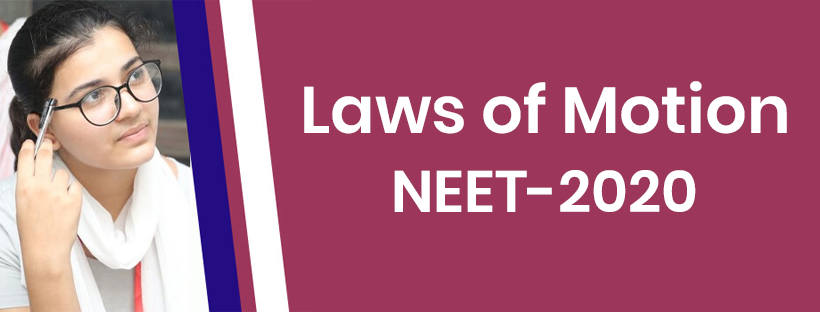Laws of Motion - NEET 2020 - Study24x7# Welcome Back

or

By Registering, you agree to our and of use.

# Laws of Motion - NEET 2020

Published on 18 September 2020The NEET 2020 exam date is near, and it is one of the most important examinations that define the career of the student. Many students are eyeing for cracking the NEET examination for the running year 2020. But before that, you need to get a clear idea of the different subtopics of the NEET 2020 syllabus under different subjects.In this article, you will cover the main topics of the fifth chapter of Physics i.e., Laws of Motion. It is a full chapter and has a lot of sections to include. So, give ample time for this section.

## Laws of Motion

Start preparing by analyzing the basics of laws of motion. The first section under the laws of motion is Force. Force is a small subsection, but you need to put a strong emphasis on it.

There are different theorems and practical formulations of the Laws of Motion, such as superposition, Lami's theorem, and others. Make sure you learn about all Newton's three laws of motion that carry the maximum weight age in the NEET examination.

Under the second subsection, learn about the fundamental forces such as weight, contact, frictional, normal, spring, and tension.

The third section that you must cover is Newton's three laws of motion and their practical implementation in the numerical. The next part is the Linear Momentum. Know the formulation of the Linear Momentum and practice various problems to be able to tackle the complexity of the questions from this section.

The next of the problems under this chapter comes with an apparent weight calculation of a body in an elevator. There are specific formulations that you need to understand precisely.

Take the help of professors or faculties to understand the concept before attempting to solve the problems. Some of the problems require combined formulations, so prefer solved papers to follow the steps and then give time on practicing the tough concepts.

The next important topic under this chapter referring to NEET 2020 syllabus is the conservation theorem of Linear Momentum. According to the previous year's questions, this section held maximum questions from this chapter and therefore it is an advice to students to put more emphasis on it.

Another critical topic is Friction, which is a prevalent topic with numerous question patterns for numerical. There are various sub-sections for Friction such as Kinetic friction, static friction and limiting friction. Take a good study material to understand the laws of friction, the coefficient, causes of different friction and others.

Some other essential points that you need to cover are centrifugal force, circular motion, pseudo force and remaining theorems for complete preparation of this section for appearing NEET 2020 examination.

These are few of the detailed subject information on the fifth chapter of Physics. If you want to be ready for the NEET 2020 examination, then refer to one of the best study materials. Take the help of your faculty to get your concepts cleared following to which your practice will play a significant role in mastering this chapter of Physics.

All The Best To All The NEET 2020 Aspirants !

Write a comment...